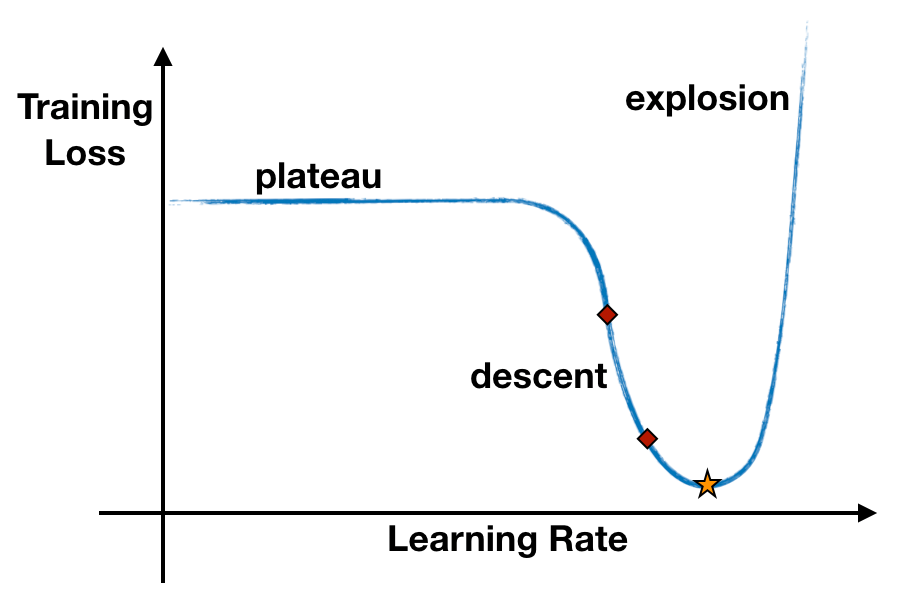# The Learning Rate Black Magic

Original article can be found here (source): Deep Learning on Medium# The Learning Rate Black Magic

Evaluation of the Learning Rate Finder Technique

The choice of the most important hyperparameter of deep models has been long considered “more an art than a science”  and relied mainly on trial and error. Indeed, one of the many challenges in training deep neural networks has historically been the selection of a good learning rate, that is until the Learning Rate Range Test (LRRT) was proposed in 2015  and made popular by the fast.ai’s deep learning library as the Learning Rate Finder (LRFinder) . In this post, we evaluate the reliability and usefulness of this technique.

Intuitively, the learning rate measures how much the model can ‘learn’ from a new mini-batch of training data, that is how much we update the model weights with information coming from each new mini-batch. The higher the learning rate, the bigger the steps we take along the trajectory to the minimum of the loss function, where the best model parameters are (Figure 1).

Learning Rate Range Test Overview

The LRRT consists of, at most, one epoch of training iterations, where the learning rate is increased at every mini-batch of data.

During the process, the learning rate goes from a very small value to a very large value (i.e. from 1e-7 to 100), causing the training loss to start with a plateau, descend to some minimum value, and eventually explode. This typical behavior can be displayed on a plot and used to select an appropriate range for the learning rate, specifically in the region where the loss is decreasing (Figure 2).

The recommended minimum learning rate is the value where the loss decreases the fastest (minimum negative gradient), while the recommended maximum learning rate is 10 times less than the learning rate where the loss is minimum.

Why not just the very minimum of the loss? Why 10 times less? Because what we actually plot is a smoothed version of the loss, and taking the learning rate corresponding to the minimum loss is likely to be too large and make the loss diverge during training.

Let’s start with reproducing one of the experiments that fast.ai published in this notebook and presented in this blog post . The experiment trains a ResNet-56 on the CIFAR-10 dataset with batch size of 512 and optimizer Stochastic Gradient Descent (SGD) with momentum. We perform the same experiment using both fast.ai LRRT implementation and a Keras implementation.

The Importance of Smoothing

First of all, the actual training loss recorded during the process should be smoothed in order for the plot to be readable and to filter out some noise. But what smoothing should we use?

A classic smoothing is an exponentially weighted average of the loss (see formula (1) and formula (2) for an unbiased version). We set the smoothing parameter β=0.98 as done in fast.ai. In some implementations, a different moving average is used, without zero as the starting value or bias correction (formula (3) below).